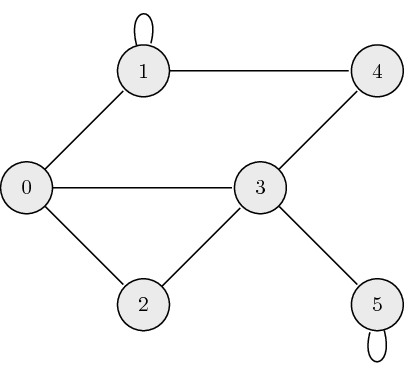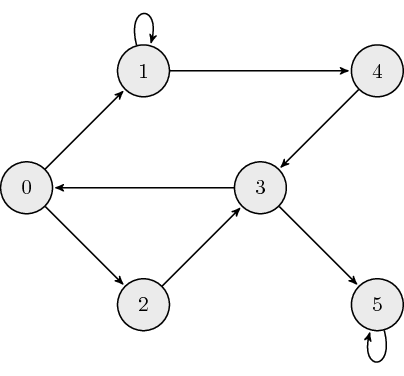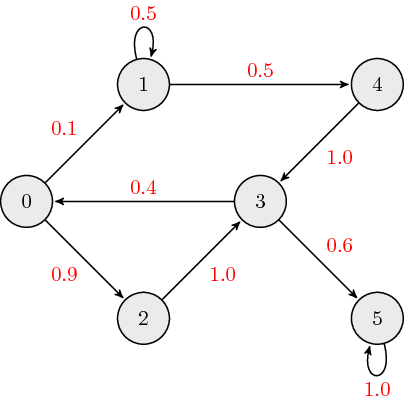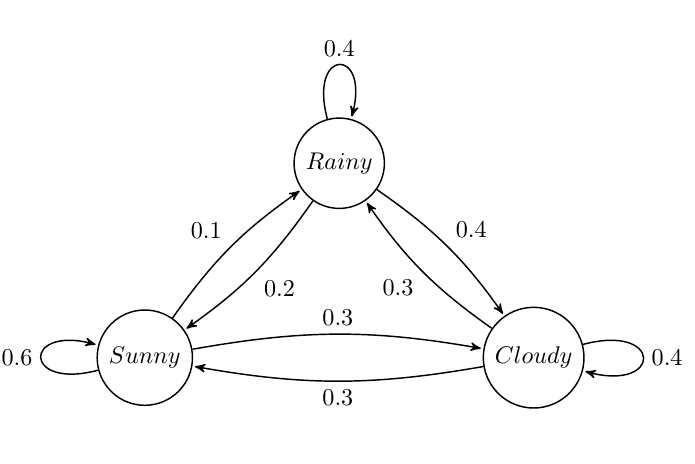# Graphs and Markov chains

## Learning Objectives

• Express a graph as a sparse matrix.
• Identify the performance benefits of a sparse matrix.

## Graphs

#### Undirected Graphs:

The following is an example of an undirected graph:The adjacency matrix, $${\bf A}$$, for undirected graphs is always symmetric and is defined as:

where $$a_{ij}$$ is the $$(i,j)$$ element of $${\bf A}$$. The adjacency matrix which describes the example graph above is:

${\bf A} = \begin{bmatrix} 0 & 1 & 1 & 1 & 0 & 0 \\ 1 & 1 & 0 & 0 & 1 & 0 \\ 1 & 0 & 0 & 1 & 0 & 0 \\ 1 & 0 & 1 & 0 & 1 & 1 \\ 0 & 1 & 0 & 1 & 0 & 0 \\ 0 & 0 & 0 & 1 & 0 & 1 \end{bmatrix}.$

#### Directed Graphs:

The following is an example of a directed graph:The adjacency matrix, $${\bf A}$$, for directed graphs is defined as:

$a_{ij} = \begin{cases} 1 \quad \mathrm{if} \ \mathrm{node}_i \leftarrow \mathrm{node}_j \\ 0 \quad \mathrm{otherwise} \end{cases},$

where $$a_{ij}$$ is the $$(i,j)$$ element of $${\bf A}$$. The adjacency matrix which describes the example graph above is:

${\bf A} = \begin{bmatrix} 0 & 0 & 0 & 1 & 0 & 0 \\ 1 & 1 & 0 & 0 & 0 & 0 \\ 1 & 0 & 0 & 0 & 0 & 0 \\ 0 & 0 & 1 & 0 & 1 & 0 \\ 0 & 1 & 0 & 0 & 0 & 0 \\ 0 & 0 & 0 & 1 & 0 & 1 \end{bmatrix}.$

#### Weighted Directed Graphs:

The following is an example of a weighted directed graph:The adjacency matrix, $${\bf A}$$, for weighted directed graphs is defined as:

$a_{ij} = \begin{cases} w_{ij} \quad \mathrm{if} \ \mathrm{node}_i \leftarrow \mathrm{node}_j \\ 0 \quad \ \ \mathrm{otherwise} \end{cases},$

where $$a_{ij}$$ is the $$(i,j)$$ element of $${\bf A}$$, and $$w_{ij}$$ is the link weight associated with edge connecting nodes $$i$$ and $$j$$. The adjacency matrix which describes the example graph above is:

${\bf A} = \begin{bmatrix} 0 & 0 & 0 & 0.4 & 0 & 0 \\ 0.1 & 0.5 & 0 & 0 & 0 & 0 \\ 0.9 & 0 & 0 & 0 & 0 & 0 \\ 0 & 0 & 1.0 & 0 & 1.0 & 0 \\ 0 & 0.5 & 0 & 0 & 0 & 0 \\ 0 & 0 & 0 & 0.6 & 0 & 1.0 \end{bmatrix}.$

Typically, when we discuss weighted directed graphs it is in the context of transition matrices for Markov chains where the link weights across each column sum to $$1$$.

## Markov Chain

A Markov chain is a stochastic model where the probability of future (next) state depends only on the most recent (current) state. This memoryless property of a stochastic process is called Markov property. From a probability perspective, the Markov property implies that the conditional probability distribution of the future state (conditioned on both past and current states) depends only on the current state.

## Markov Matrix

A Markov/Transition/Stochastic matrix is a square matrix used to describe the transitions of a Markov chain. Each of its entries is a non-negative real number representing a probability. Based on Markov property, next state vector $${\bf x}_{k+1}$$ is obtained by left-multiplying the Markov matrix $${\bf M}$$ with the current state vector $${\bf x}_k$$.

${\bf x}_{k+1} = {\bf M} {\bf x}_k$

In this course, unless specifically stated otherwise, we define the transition matrix $${\bf M}$$ as a left Markov matrix where each column sums to $$1$$.

Note: Alternative definitions in outside resources may present $${\bf M}$$ as a right markov matrix where each row of $${\bf M}$$ sums to $$1$$ and the next state is obtained by right-multiplying by $${\bf M}$$, i.e. $${\bf x}_{k+1}^T = {\bf x}_k^T {\bf M}$$.

A steady state vector $${\bf x}^*$$ is a probability vector (entries are non-negative and sum to $$1$$) that is unchanged by the operation with the Markov matrix $$M$$, i.e.

${\bf M} {\bf x}^* = {\bf x}^*$

Therefore, the steady state vector $${\bf x}^*$$ is an eigenvector corresponding to the eigenvalue $$\lambda=1$$ of matrix $${\bf M}$$. If there is more than one eigenvector with $$\lambda=1$$, then a weighted sum of the corresponding steady state vectors will also be a steady state vector. Therefore, the steady state vector of a Markov chain may not be unique and could depend on the initial state vector.

## Markov Chain Example

Suppose we want to build a Markov Chain model for weather predicting in UIUC during summer. We observed that:

• a sunny day is $$60\%$$ likely to be followed by another sunny day, $$10\%$$ likely followed by a rainy day and $$30\%$$ likely followed by a cloudy day;
• a rainy day is $$40\%$$ likely to be followed by another rainy day, $$20\%$$ likely followed by a sunny day and $$40\%$$ likely followed by a cloudy day;
• a cloudy day is $$40\%$$ likely to be followed by another cloudy day, $$30\%$$ likely followed by a rainy day and $$30\%$$ likely followed by a sunny day.

The state diagram is shown below:The Markov matrix is

${\bf M} = \begin{bmatrix} 0.6 & 0.2 & 0.3 \\ 0.1 & 0.4 & 0.3 \\ 0.3 & 0.4 & 0.4 \end{bmatrix}.$

If the weather on day $$0$$ is known to be rainy, then

${\bf x}_0 = \begin{bmatrix} 0 \\ 1 \\ 0 \end{bmatrix};$

and we can determine the probability vector for day $$1$$ by

${\bf x}_1 = {\bf M} {\bf x}_0.$

The probability distribution for the weather on day $$n$$ is given by

${\bf x}_n = {\bf M}^{n} {\bf x}_0.$